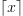/NumPy 1.17

# numpy.ceil

`numpy.ceil(x, /, out=None, *, where=True, casting='same_kind', order='K', dtype=None, subok=True[, signature, extobj]) = <ufunc 'ceil'>`

Return the ceiling of the input, element-wise.

The ceil of the scalar `x` is the smallest integer `i`, such that `i >= x`. It is often denoted as.

Parameters: `x : array_like` Input data. `out : ndarray, None, or tuple of ndarray and None, optional` A location into which the result is stored. If provided, it must have a shape that the inputs broadcast to. If not provided or `None`, a freshly-allocated array is returned. A tuple (possible only as a keyword argument) must have length equal to the number of outputs. `where : array_like, optional` This condition is broadcast over the input. At locations where the condition is True, the `out` array will be set to the ufunc result. Elsewhere, the `out` array will retain its original value. Note that if an uninitialized `out` array is created via the default `out=None`, locations within it where the condition is False will remain uninitialized. **kwargs For other keyword-only arguments, see the ufunc docs. `y : ndarray or scalar` The ceiling of each element in `x`, with `float` dtype. This is a scalar if `x` is a scalar.

See also

#### Examples

```>>> a = np.array([-1.7, -1.5, -0.2, 0.2, 1.5, 1.7, 2.0])
>>> np.ceil(a)
array([-1., -1., -0.,  1.,  2.,  2.,  2.])
```

© 2005–2019 NumPy Developers
Licensed under the 3-clause BSD License.
https://docs.scipy.org/doc/numpy-1.17.0/reference/generated/numpy.ceil.html